Next: Discussions Up: Results Previous: Nonlinearly active modes and

## Correlations of phases, phase factors and amplitudes.

WT closure relies on the RPA properties of the wave fields, i.e. that the amplitudes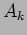and the phase factors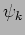are statistically independent variables. On the other hand, WT calculation for the phases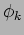shows that these quantities get correlated. In order to check these properties and predictions numerically, let us introduce a function that measures the degree of statistical dependence (or independence) of some Fourier-space variables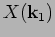and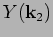,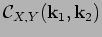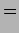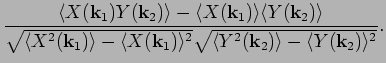(31)

For example, we can examine to what degree amplitudes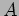and independent of the phase factors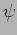by looking at the function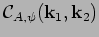for different values of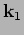and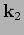. Independence of the amplitudes at different wavenumbers can be examined by the auto-correlation function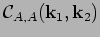, and similar for the phase factors and the phases. We restrict ourselves with choosing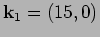and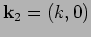with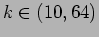. Figure 15 shows the values of correlators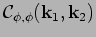and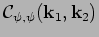as functions of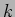. In agreement with WT predictions, auto-correlations of's are very small whereas the ones of's are significant (except, of course, for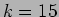where by definition these correlators are equal to one). Correlatorsandare shown in figure 16. Again, we see a good agreement with the WT prediction: these correlations are very small (except, again,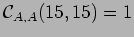).

 [width=10cm]Psi_psi_corr.eps
 [width=10cm]A_psi_corr.epsNext: Discussions Up: Results Previous: Nonlinearly active modes and
Dr Yuri V Lvov 2007-01-16